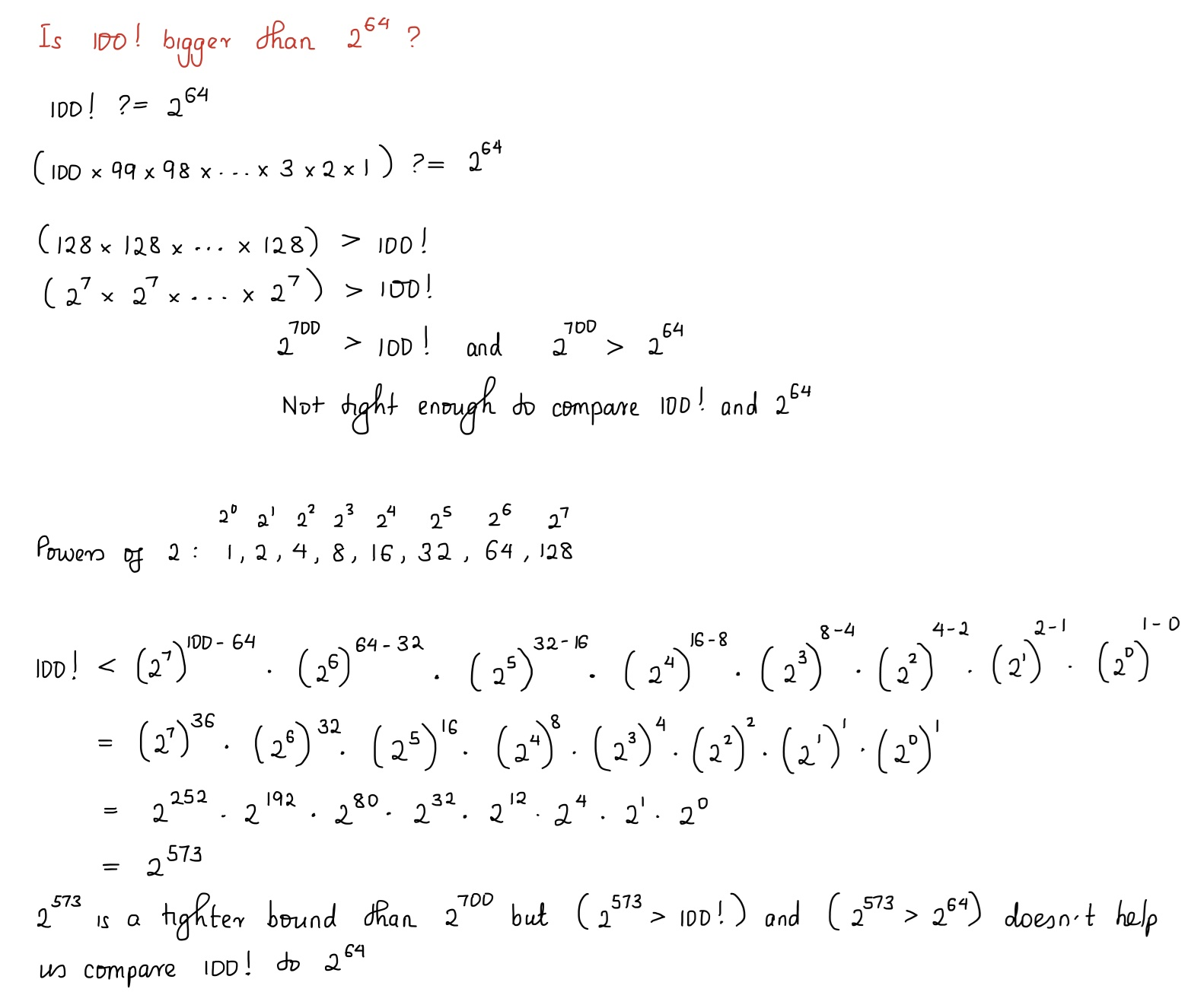# 020. Factorial Digit Sum

## Problem Statement

$$n!$$ means $$n \times (n - 1) \times … \times 3 \times 2 \times 1$$.

For example, $$10! = 10 \times 9 \times … \times 3 \times 2 \times 1 = 3628800$$, and the sum of the digits in the number $$10!$$ is $$3 + 6 + 2 + 8 + 8 + 0 + 0 = 27$$.

Find the sum of the digits in the number $$100!$$

## My Solution

It doesn’t look like we can do without calculating the actual value of $$100!$$, and that is something that is too burdensome to compute by hand. Programming it is!

How big is $$100!$$? Most programming languages have built-in support for numbers less than $$2^{64} - 1$$.Failed attempt at comparing $$2^{64}$$ to $$100!$$

Using Stirling’s approximation, $$\log_{k}(n!) \approx n \log_{k}(n) - n \cdot \log_{k}(e)$$, determines that $$n!$$ starts outgrowing $$2^{n}$$ when $$n \approx e \cdot k$$. In practice tend to use $$n \gg e \cdot k$$, so we assume that factorials grow faster than exponentials.

In our case, $$100 \gg 2 \cdot e$$, and so the most convenient language for this problem is one that has large integer support, e.g. Python.

>>> from math import factorial
>>> s = f"{factorial(100)}"
>>> sum(int(i) for i in s)
648


But what about Lisp? I’ve seen it enough times on Hacker News to want to toy with it. Common-Lisp.net seems like a good starting point. Portacle , the recommended beginner-friendly way to run Lisp, does not work for me despite trying these workarounds . has some alternate instructions.

Common Lisp is a standard, and Steel Bank Common Lisp (SBCL) is a popular Common Lisp implementation. Quicklisp is a package manager. SLIME: The Superior Lisp Interaction Mode for Emacs is a popular Common Lisp IDE built on Emacs. These components might not have been as apparent if Portacle worked as expected. Furthermore, I took less time to get my setup via this “longer” route.

Mistakenly did brew install emacs instead of brew install --cask emacs as advocated for by GNU Emacs download - GNU Project . homebrew-cask extends Homebrew by installing and managing GUI macOS applications .

Emacs has an emphasis on being productive from the keyboard, e.g. providing alternatives to using the arrow keys to avoid leaving the touch typing position. However, I still like VS Code because of the extensions that I have. Presumably, once I customize Emacs in a similar way, I’ll move into the Emacs camp and join the eternal Emacs vs. Vim wars. Emacs Keymap provides Emacs keyboard shortcuts in VS Code.

has a nice video of setting up Common Lisp, Emacs, Slime and Quicklisp such that we can iterate on the code efficiently. I think that’s pretty cool. Python’s REPL is the closest that I’ve come to such a development environment.

#|
Common Lisp objects have types, while variables do not.
https://lispcookbook.github.io/cl-cookbook/type.html
|#

(defun factorial (n)
"Return the factorial of n."
; Return statements don't need to be explicit. The result of the last
; evaluated expression is returned.
(if (= n 1)
n
(* n
(factorial (- n 1)))))

(defun sum_of_digits (n)
"Return the sum of the digits in n."
#|
* https://lispcookbook.github.io/cl-cookbook/strings.html
* https://lispcookbook.github.io/cl-cookbook/iteration.html
|#
(loop for c across (write-to-string n)
sum (parse-integer (string c)) into total

(defun project_euler_020 ()
"Solve https://projecteuler.net/problem=20"
(write (sum_of_digits (factorial 100))))



Emacs + Slime has a steep learning curve. The keyboard bindings are unlike anything that I frequently use. However, I think we did use either Vim or Emacs in COS 217, because C-x C-s to save a file feels familiar.

First impressions of Lisp development: the code is quite readable. Pleasantly surprised by sum (parse-integer (string c)) into total.

## Learning from Others' Solutions

I could have gone without the integer to string to characters to integers roundtrip, e.g.

def sum_of_digits(n):
total = 0
while (n > 0):
total += n % 10
n = int(n / 10)

1. Which function grows faster, exponential or factorial? - Stack Overflow.stackoverflow.com . Accessed Jan 16, 2022.
2. Installing Common Lisp, Emacs, Slime & Quicklisp - YouTube.www.youtube.com . Accessed Jan 16, 2022.